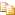﻿Concat Method (left, right)AvalonEdit
Concat Method (left, right)
NamespacesICSharpCode.AvalonEdit.UtilsRope<(Of <(<'T>)>)>Concat(Rope<(Of <<'(T>)>>), Rope<(Of <<'(T>)>>))
Concatenates two ropes. The input ropes are not modified. Runs in O(lg N + lg M).Declaration Syntax
 C# Visual Basic Visual C++
```public static Rope<T> Concat(
Rope<T> left,
Rope<T> right
)```
```Public Shared Function Concat ( _
left As Rope(Of T), _
right As Rope(Of T) _
) As Rope(Of T)```
```public:
static Rope<T>^ Concat(
Rope<T>^ left,
Rope<T>^ right
)```Parameters
left (Rope<(Of <(<'T>)>)>)
right (Rope<(Of <(<'T>)>)>)Remarks
This method counts as a read access and may be called concurrently to other read accesses.

Assembly: ICSharpCode.AvalonEdit (Module: ICSharpCode.AvalonEdit.dll) Version: 5.0.0.4238